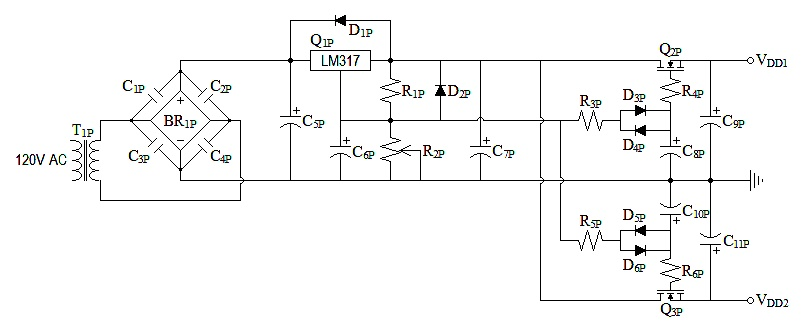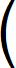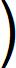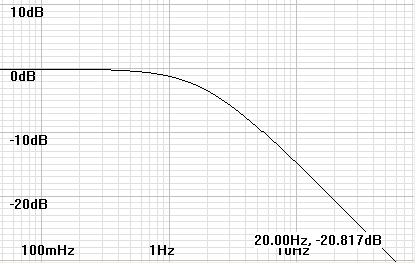Home  │ Audio Home Page

Updated March 11, 2010. See Document History at end for details.

## Line Level Class A Power Supply

This is an example only.  It is not intended to be final or complete design.

Figure 1:  SchematicParts List T1P 20V transformer (or somewhat higher if 20V unavailable) BR1P bridge rectifier, peak current specification exceeding peak transformer current. C1P-C4P 0.1µF ceramic C5P 4700µF, 35V electrolytic Q1P LM317 D1P,D2P 1N4002 diodes C6P 10µF, 35V electrolytic R1P 240Ω, 5%
 R2P 5kΩ or 10kΩ pot C7P 100µF, 35V electrolytic Q2P,Q3P IRF510 n-ch mosfet D3P-D6P 1N914 or 1N4148 diodes R3P,R5P 4.7kΩ, 5% R4P,R6P 1kΩ, 5% C8P,C10P 220µF, 35V electrolytic C9P 10,000µF, 35V electrolytic C11P 3300µF, 35V electrolytic
These specific calculations are for a power supply for the circuit of JFET Phono Preamp - Active Inductor Example.  You may have to change some values for other applications.  See text.for calculations.

#### Initial Design Decisions

• The power supply is designed to be simple source follower type for class-a friendly output impedance.
• Prefiltering is by LM317 for better line regulation.  LM317 circuit design is as recommended by datasheet.
• Unique design element pertains to adding parallel diodes to gate circuit of source follower.  The diodes create a variable RC constant.  This provides for quick charge through the resistor to nearly the desired gate voltage then a slow increase to an enormous RC time constant to hold a constant gate voltage.
• Load requirements:  27.9778112mA out of VDD1, 19.4563897mA out of VDD2.

#### Calculations

Some of the component choices are not very critical, but I chose to calculate these.
• Calculate transformer specification.
vTRANSFORMER = 0.7071 x (vSUPPLY + vgs-max-Q2 + vDROPOUT-LM317 + vDIODE-DROP) = 0.7071 x (18V + 4V + 3V + 1V)  = 18.3846V
Round up to standard value of 20V.
• Calculate C5P for  ripple of 0.5V.
Refer article: Power Supply Ripple Calculations and Capacitor Size, equation (3).
iLM317-INPUT = iDD1 + iDD2 + iR1P + iADJ-LM317 = 27.9778112mA + 19.4563897mA + 5mA + 100µA = 52.5342009mA
 C5P = i fΔv = 52.5342009mA 60Hz x 0.5V = 1.75114003mF
Choose instead common larger value of 4700µF
• Choose R3P,R5P and C8P,C10P for a time constant of one second.
I chose these values a good while ago to meet this specification.  How I chose the first component in order to calculate the second, I do not remember.  Perhaps it was a noise consideration.  Calculate total noise from capacitor noise equation.  (The capacitor is not the source of the noise itself but interacts with the resistor in a way to become the sole determining factor.)
 vNOISE-C8P-TOTAL = sqrtkT C= sqrt1.3806504e-23 x 298.15ºK 220µF= 4.325615651nV
R3P = 1/C8P = 4.545454545kΩ, rounded up to nearest 5% standard value is 4.7kΩ.

As a matter of curiosity, the 1N914 specification of 5nA reverse current at 20V suggests an ac impedance of 800MΩ at very low ac voltages.  (This is due to leakage resistance.  Ideal calculated reverse current is very much lower.)  220µF x 400MΩ gives a settled time constant of 88,000  seconds.  Because this is such a large value, I suspect it to be very imprecise.
• Establish lower boundary for C9P,C11P based on these criteria:
The common source circuit supplied by VDD1 is very sensitive to its power supply.  Indeed, the power supply is in the signal path for this stage.  Maximum output current from VDD1 is 41.4651312mA. The gfs of IRF510 is 1.3S @ 3.4A.  I want primarily capacitive power supply output impedance.  The transistor output impedance is non-linear (although with nice euphonic 2nd order harmonics!) and with the capacitor value determines the pole above which output impedance becomes capacitive.  Initially try for a pole at 2Hz.

IRF510 specification:  gfs = 1.3S @ 3.4A.

Calculate MOSFET constant from gfs@iD  specification.
 kn = gfs2 4iD = 1.3S2 4(3.4A) = 124.2647059mA/V2

Recalculate gfs for specific iD.
gfs = 2 x sqrt(kniD) = 2 x sqrt(124.2647059mA/V2 x 27.9778112mA) = 117.9263241mS

 Rout-Q2P = 1 gfs = 1 2 x sqrt(kniD) = 1 2 x sqrt(117.9263241mS x 27.9778112mA) = 8.70477913Ω

 C9P = 1 2πfpoleRout-Q2P = 1 2π x 2Hz x 8.70477913Ω = 9.14181398mF

Choose C9P = 10,000µF and solve for the pole frequency.
 fpole = 1 2πC9PRout-Q2P = 1 2π x 10mF x 8.70477913Ω = 1.828362796Hz

 Figure 2:  Bode plot representing suppression of MOSFET non-linearity by chosen bypass capacitor of 10,000µFA more common choice of 1000µF gives a pole frequency of 18.28362796Hz.  This choice may give a slight increase in low bass distortion, and perhaps why some reviewers hear mid-bass bloom in some class A equipment.

VDD2 drives a circuit (source follower) which is relatively insensitive to power supply fluctuations.  Therefore you can choose a more arbitrary value here.  Save money here to buy a larger C9P.
I choose  C11P = 3300µF.

Document History

January 5, 2010 Created
January 5, 2010 Replace vague load specifications with exact ones from article JFET Phono Preamp - Active Inductor Example and update calculations.
March 11, 2010.  Corrected for improper gfs presumptions.